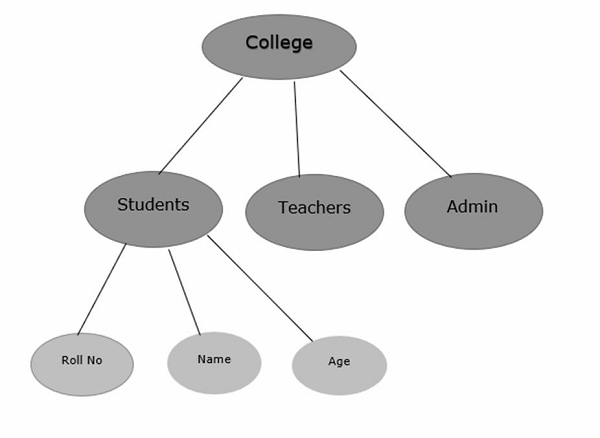# What is Hierarchical model in DBMS?

Data Models in the Database Management System (DBMS)are divided into three categories. These are as follows −

• Object Model − It uses concepts like entities, attributes, and their relationship. This model can be used to describe the data at the conceptual and external level. For Example: E-R model.

• Physical Model − These models describe how the data is stored in the computer. This model is used to describe the data at the internal level.

• Record based logical models − These models are used in describing the data at the logical and view level. It is used to specify the overall logical structure of the database.

## Features of Hierarchical Model

The features of hierarchical model are as follows −

• It is based on tree structure.

• It consists of a collection of records that are connected to each other by links.

• The tree structure used in hierarchical models is called as routed true.

• The root node of that tree is an empty node.

• So, Hierarchical model is a collection of routed trees and the relationship that exists in the hierarchical model is one to many and one to one.

The advantages of hierarchical model are as follows −

• It is easy to understand.

• More efficient than the ER model.

The disadvantages of hierarchical model are as follows −

• Data inconsistency occurs when the parent node is deleting that result in the deletion of the child node.

• Wastage of storage space.

• Complex to design.

• Absence of structural independence.

Given below is an example of the hierarchical model in pictorial format −